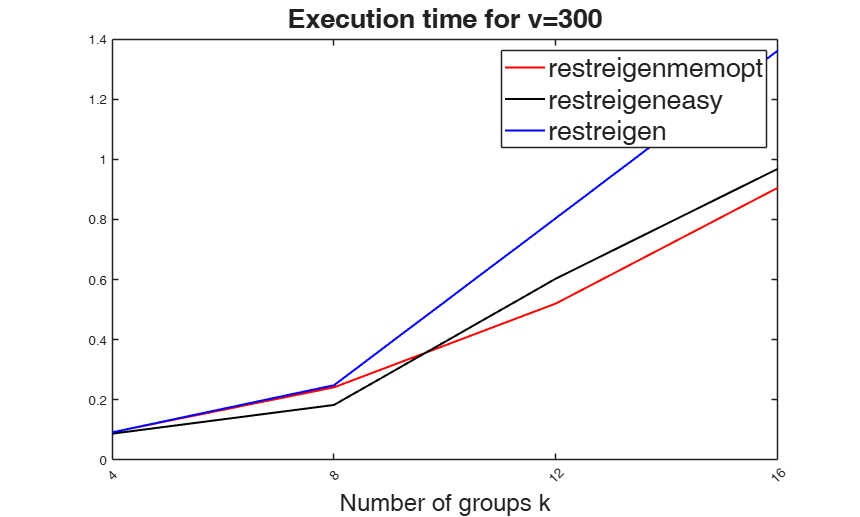restreigeneasy

restreigeneasy computes eigenvalues restriction (without Dykstra algorithm)

Syntax

• out=restreigeneasy(eigenvalues, niini, restr)example
• out=restreigeneasy(eigenvalues, niini, restr, tol)example

Description

restreigeneasy restricts the eigenvalues according to the constraint specified in scalar restr. This function is a readable and understandble (but sometimes slower) version of routine restreigen.

out =restreigeneasy(eigenvalues, niini, restr) Compiting time of three implementations of the eigenvalues restriction.

out =restreigeneasy(eigenvalues, niini, restr, tol) Example using all default options.

Examples

expand allCompiting time of three implementations of the eigenvalues restriction.

vmin  = 300; vmax  = 300; vstep = 1; vv = vmin:vstep:vmax;
kmin  = 4;   kmax  = 16;  kstep = 4; kk = kmin:kstep:kmax;
cycles = 1;
t=zeros(floor((vmax-vmin)/vstep),length(kk)); t_easy=t; t2_easy=t;
ik=0;
for k = kk
ik=ik+1;
iv=0;
for v=vv
disp(['p=',num2str(v),' - k=',num2str(k)]);
iv = iv+1;
eigenvalues=abs(10*randn(v,k));
niini=randi(1000,k,1);
t0=tic;
for s=1:cycles, out  = restreigen(eigenvalues,niini,1.1,1e-8);end
t(iv,ik) = toc(t0);
t1=tic;
for s=1:cycles, out1 = restreigenmemopt(eigenvalues,niini,1.1,1e-8);end
t_easy(iv,ik)=toc(t1);
t2=tic;
for s=1:cycles, out2 = restreigeneasy(eigenvalues,niini,1.1,1e-8);end
t2_easy(iv,ik)=toc(t2);
if sum(sum(out2-out1))>0
disp('Zob!');
end
end
end
figure;
vvv = 1;
h2=plot(1:ik,t_easy(vvv,:),'-r',1:ik,t2_easy(vvv,:),'-k',1:ik,t(vvv,:),'-b');
set(h2,'LineWidth',1.5);
legend('restreigenmemopt' , 'restreigeneasy', 'restreigen','FontSize',20);
xlabel('Number of groups k','FontSize',18);
set(gca,'Xtick',1:ik,'XtickLabel',kk,'XTickLabelRotation',45);
title(['Execution time for v=' num2str(vv(vvv))],'FontSize',20);
p=300 - k=4
p=300 - k=8
p=300 - k=12
p=300 - k=16Example using all default options.

Suppose v=3 and k=4 so the matrix containing the eigenvalues is 3-by-4 First column of matrix eigenvalues contains the eigenvalues of the first group Second column of matrix eigenvalues contains the eigenvalues of the second group Thrid column of matrix eigenvalues contains the eigenvalues of the third group Fourth column of matrix eigenvalues contains the eigenvalues of the fourth group

rng(10,'twister')
eigenvalues=abs(10*randn(3,4));
% niini is the vector containing the sizes of the 4 groups
niini=[30;40;20;10];
out=restreigeneasy(eigenvalues,niini,1.1)
disp('Input matrix of unrestricted eigenvalues')
disp(eigenvalues)
disp('Output matrix of restricted eigenvalues')
disp(out)
disp('Ratio between largest and smallest unrestricted eigenvalues')
disp(max(max(eigenvalues))/min(min(eigenvalues)))
disp('Ratio between largest and smallest restricted eigenvalues')
disp(max(max(out))/min(min(out)))

Related Examples

expand all

Compare speed.

We compare the speed of restreigneasy with that of restreigen. We use userepmat=2 if the current MATLAB version if >=R2017a or userepmat =1 if MATLAB version is >=R2013a but <R2017a else we use userepmat =0

v=10;
k=8;
tol=1e-8;
if verLessThanFS(9.2)== false
% If MATLAB version is at least 2017a
userepmat=2;
elseif verLessThanFS(8.1) == false
% if MATLAB version is at least R2013b
userepmat=1;
else
userepmat=0;
end
oldroutinetime=0;
newroutinetime=0;
rng(1)
for j=1:10000
eigenvalues=100*abs(randn(v,k));
% niini is the vector containing the sizes of the 4 groups
niini=randi(100,[k,1]);
tic;
outold=restreigeneasy(eigenvalues,niini,1.1);
% Uncomment the line below if you want
% outold=restreigen(eigenvalues,niini,1.1,tol,1);
oldroutinetime=oldroutinetime+toc;
tic;
outnew=restreigen(eigenvalues,niini,1.1,tol,userepmat);
newroutinetime=newroutinetime+toc;
if max(max(abs(outold-outnew)))>1e-5
error('The two routines are different')
end
end
disp(['Computing time of restreigeneasy: ' num2str(oldroutinetime)])
disp(['Computing time of restreigen: ' num2str(newroutinetime)])

Input Arguments

eigenvalues — Eigenvalues. Matrix.

v x k matrix containing the eigenvalues of the covariance matrices of the k groups.

v is the number of variables of the dataset which has to be clustered.

Data Types: single| double

niini — Cluster size. Vector.

k x 1 vector containing the size of the k clusters

Data Types: single| double

restr — Restriction factor. Scalar.

Scalar containing the restr parameter in tclust program.

More in detail, parameter restr defines the cluster's shape restrictions, which are applied on all clusters during each iteration.

Setting restr to 1, yields the strongest restriction, forcing all eigenvalues/determinants to be equal and so the method looks for similarly scattered (respectively spherical) clusters.

Data Types: single| double

tol — tolerance. Scalar defining the tolerance of the procedure.

The default value is 1e-8

Example: 'tol',[1e-18]

Data Types: double

Output Arguments

out —Restricted eigenvalues. Matrix

v-by-k matrix containing restricted eigenvalues.

The ratio between two possible elements in matrix out is not greater than restr

Fritz H., Garcia-Escudero, L.A. and Mayo-Iscar, A. (2013), A fast algorithm for robust constrained clustering, "Computational Satistics and Data Analysis", Vol. 61, pp. 124-136.